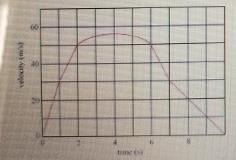# Problem: The graph in the figure (Figure 1) shows the velocity v of a sports car as a function of time t (a) Find the maximum acceleration amax of the car. Express your answer in meters per second per second to the nearest integer.(b) Find the minimum magnitude of the acceleration amin of the car

###### FREE Expert Solution

Acceleration:

$\overline{){\mathbf{a}}{\mathbf{=}}\frac{\mathbf{∆}\mathbf{v}}{\mathbf{∆}\mathbf{t}}}$

The slope of a velocity time graph is the acceleration.

99% (34 ratings)###### Problem Details

The graph in the figure (Figure 1) shows the velocity v of a sports car as a function of time t(a) Find the maximum acceleration amax of the car. Express your answer in meters per second per second to the nearest integer.

(b) Find the minimum magnitude of the acceleration amin of the car